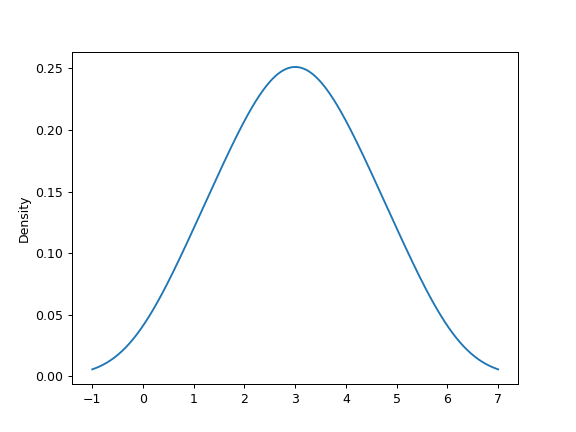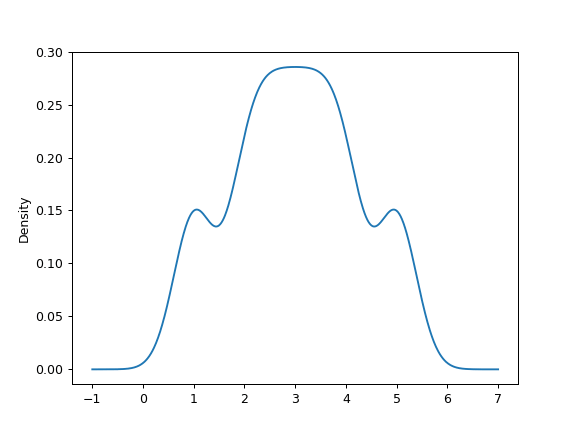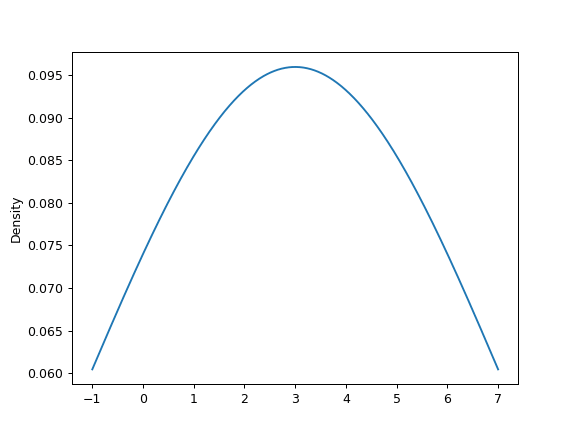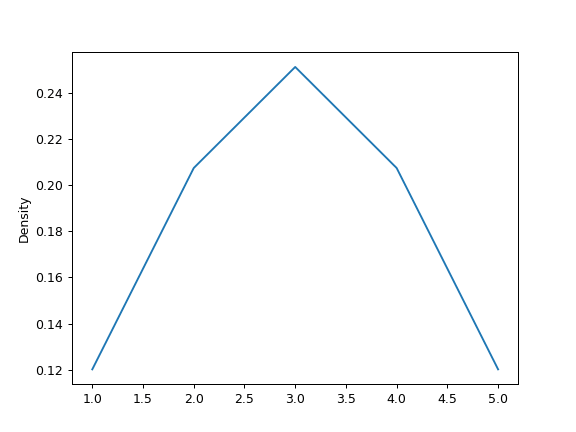/pandas 0.23

# pandas.Series.plot.density

`Series.plot.density(bw_method=None, ind=None, **kwds)` [source]

Generate Kernel Density Estimate plot using Gaussian kernels.

In statistics, kernel density estimation (KDE) is a non-parametric way to estimate the probability density function (PDF) of a random variable. This function uses Gaussian kernels and includes automatic bandwith determination.

Parameters: bw_method : str, scalar or callable, optional The method used to calculate the estimator bandwidth. This can be ‘scott’, ‘silverman’, a scalar constant or a callable. If None (default), ‘scott’ is used. See `scipy.stats.gaussian_kde` for more information. ind : NumPy array or integer, optional Evaluation points for the estimated PDF. If None (default), 1000 equally spaced points are used. If `ind` is a NumPy array, the KDE is evaluated at the points passed. If `ind` is an integer, `ind` number of equally spaced points are used. **kwds : optional Additional keyword arguments are documented in `pandas.Series.plot()`. `axes : matplotlib.axes.Axes or numpy.ndarray of them`

See also

`scipy.stats.gaussian_kde`
Representation of a kernel-density estimate using Gaussian kernels. This is the function used internally to estimate the PDF.
`DataFrame.plot.kde`
Generate a KDE plot for a DataFrame.

#### Examples

Given a Series of points randomly sampled from an unknown distribution, estimate its PDF using KDE with automatic bandwidth determination and plot the results, evaluating them at 1000 equally spaced points (default):

```>>> s = pd.Series([1, 2, 2.5, 3, 3.5, 4, 5])
>>> ax = s.plot.kde()
```A scalar bandwidth can be specified. Using a small bandwidth value can lead to overfitting, while using a large bandwidth value may result in underfitting:

```>>> ax = s.plot.kde(bw_method=0.3)
``````>>> ax = s.plot.kde(bw_method=3)
```Finally, the `ind` parameter determines the evaluation points for the plot of the estimated PDF:

```>>> ax = s.plot.kde(ind=[1, 2, 3, 4, 5])
```© 2008–2012, AQR Capital Management, LLC, Lambda Foundry, Inc. and PyData Development Team
Licensed under the 3-clause BSD License.
http://pandas.pydata.org/pandas-docs/version/0.23.4/generated/pandas.Series.plot.density.html